Open in App
Not now

# Write custom aggregation function in Pandas

• Last Updated : 20 Aug, 2020

Pandas in python in widely used for Data Analysis purpose and it consists of some fine data structures like Dataframe and Series. There are several functions in pandas that proves to be a great help for a programmer one of them is an aggregate function. This function returns a single value from multiple values taken as input which are grouped together on certain criteria. A few of the aggregate functions are average, count, maximum, among others.

Syntax: DataFrame.agg(func=None, axis=0, *args, **kwargs)

Parameters:

• axis: {0 or ‘index’, 1 or ‘columns’} = 0 or ‘index’ means the function is applied to each column and 1 or ‘columns’ means the function is applied to each row.
• func: function, str, list or dict = It describes the function that is to be used for aggregation. Accepted combinations are: function, string function name (str), list of functions (list/dict).
• *args: It specifies the positional arguments to pass to the function.
• **kwargs: It specifies the keyword arguments to pass to the function.

Return: This function can return scalar, Series or Dataframe. The return is scalar when Series.agg is called with a single function, it is Series when Dataframe.agg is called with a single function, it will be Dataframe when Dataframe.agg is called with several functions.

Let’s create a Dataframe:

## Python3

 `# import pandas library``import` `pandas as pd`` ` `# create a Dataframe``df ``=` `pd.DataFrame([[``10``, ``20``, ``30``],``                  ``[``40``, ``50``, ``60``],``                  ``[``70``, ``80``, ``90``],``                  ``[``100``,``110``,``120``]],``                 ``columns``=``[``'Col_A'``, ``'Col_B'``,``                          ``'Col_C'``])``# show the dataframe``df`

Output: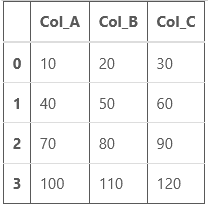Now, let’s perform some operations:

1. Performing aggregation over the rows: This performs aggregate functions over the rows of the Dataframe. As you can see in the below examples, the example 1 has two keywords inside the aggregate function, sum and min. The sum adds up the first (10,40,70,100), second (20,50,80,110) and third (30,60,90,120) element of each row separately and print it, the min finds the minimum number among the elements of rows and print it. Similar process is with the second example.

Example 1:

## Python3

 `df.agg([``'sum'``, ``'min'``])`

Output: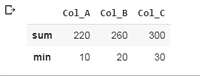Example 2:

## Python3

 `df.agg([``'sum'``, ``'min'``, ``'max'``])`

Output: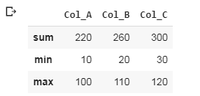2. Performing aggregation per column: This performs aggregate function on the columns, the columns are selected particularly as shown in the examples. In the first example, two columns are selected, ‘Col_A’ and ‘Col_B’ and operations are to be performed on them. For Col_A, the minimum value and the summed up value is calculated and for the Col_B, minimum and maximum value is calculated. Similar process is with example 2.

Example 1:

## Python3

 `df.agg({``'Col_A'` `: [``'sum'``, ``'min'``], ``        ``'Col_B'` `: [``'min'``, ``'max'``]})`

Output: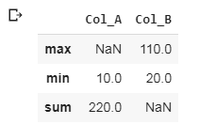Example 2:

## Python3

 `df.agg({``'Col_A'` `: [``'sum'``, ``'min'``],``        ``'Col_B'` `: [``'min'``, ``'max'``],``        ``'Col_C'` `: [``'sum'``, ``'mean'``]})`

Output: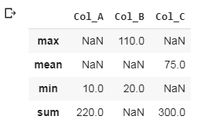Note: It will print NaN if a particular aggregation is not performed on a particular column.

3. Performing aggregation over the columns: This performs aggregate function over the columns. As shown in example 1, the mean of first (10,20,30), second (40,50,60), third (70,80,90) and fourth (100,110,120) elements of each column is calculated separately and printed.

Example:

## Python3

 `df.agg(``"mean"``, axis ``=` `"columns"``)`

Output: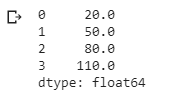4. Custom Aggregate function: Sometimes it becomes a need to create our own aggregate function.

Example: Consider a data frame consisting of student id (stu_id), subject code (sub_code) and marks (marks).

## Python3

 `# import pandas library``import` `pandas as pd``  ` `# Creating DataFrame``df ``=` `pd.DataFrame(``    ``{``'stud_id'` `: [``101``, ``102``, ``103``, ``104``, ``                  ``101``, ``102``, ``103``, ``104``],``     ``'sub_code'` `: [``'CSE6001'``, ``'CSE6001'``, ``'CSE6001'``, ``                   ``'CSE6001'``, ``'CSE6002'``, ``'CSE6002'``,``                   ``'CSE6002'``, ``'CSE6002'``],``     ``'marks'` `: [``77``, ``86``, ``55``, ``90``, ``                ``65``, ``90``, ``80``, ``67``]}``)`` ` `# Printing DataFrame``df`

Output: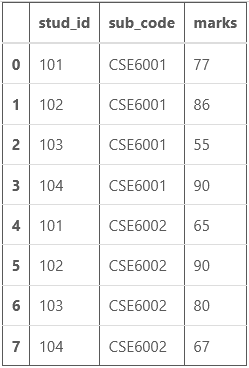Now if you need to calculate the total marks (marks of two subjects) of each student (unique stu_id). This process can be done using custom aggregate function. Here my custom aggregate function is ‘total’.

## Python3

 `# Importing reduce for ``# rolling computations``from` `functools ``import` `reduce`` ` `# define a Custom aggregation ``# function for finding total``def` `total(series):``      ``return` `reduce``(``lambda` `x, y: x ``+` `y, series)`` ` `# Grouping the output according to ``# student id and printing the corresponding ``# total marks and to check whether the``# output is correct or not, sum function ``# is also used to print the sum.``df.groupby(``'stud_id'``).agg({``'marks'``: [``'sum'``, total]})`

Output: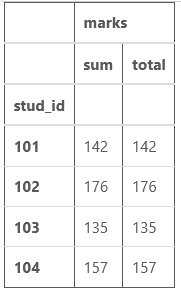As you can see, both the columns have same values of total marks, so our aggregate function is correctly calculating the total marks in this case.

My Personal Notes arrow_drop_up